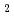※ 半角英数字
年 ～
年

# Estimation for mass transfer coefficient under two-phase flow conditions using two gas components

## 2気体成分を用いた二相流条件下における物質移動係数の推定

For proper source term evaluation, we constructed the theoretical model to estimate the mass transfer coefficient of gaseous iodine species under two-phase flow conditions, which complicates the direct experimental measurements. The mass transfer speed is determined by the product of the overall mass transfer coefficient and the interfacial area. By using the ratio of two gas components, the interfacial area, which is an important parameter that is difficult to measure, can be canceled out and the ratio of their overall mass transfer coefficients can be obtained. This ratio is expected to be equal to the ratio of their diffusion coefficients. Therefore, the unknown mass transfer coefficient such as iodine species can be estimated using the diffusion coefficients of two gas components and the reference mass transfer coefficient such as O. We carried out the experiments using the bubble column to confirm this relationship. From the results in this study, we confirmed that the ratio of the overall mass transfer coefficient was in good agreement with the ratio of diffusion coefficient under the bubbly flow conditions. Using this relationship confirmed in this study, we estimated the mass transfer coefficient of I, one of the iodine species.

Access Altmetrics : - Accesses :
• 登録番号 : AA20210908
• 論文投稿番号 :

[CLARIVATE ANALYTICS], [WEB OF SCIENCE], [HIGHLY CITED PAPER & CUP LOGO] and [HOT PAPER & FIRE LOGO] are trademarks of Clarivate Analytics, and/or its affiliated company or companies, and used herein by permission and/or license.